# Olympiad Test: Square And Square Roots

## 20 Questions MCQ Test Mathematical Olympiad Class 8 | Olympiad Test: Square And Square Roots

Description
Attempt Olympiad Test: Square And Square Roots | 20 questions in 40 minutes | Mock test for Class 8 preparation | Free important questions MCQ to study Mathematical Olympiad Class 8 for Class 8 Exam | Download free PDF with solutions
QUESTION: 1

### What is the value of x if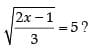Solution:

Given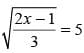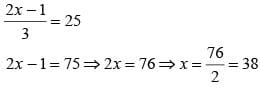QUESTION: 2

### What is the greatest number of five digits which is a perfect square?

Solution:

The greatest number of five digits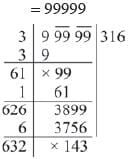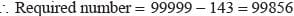QUESTION: 3

### What is the least number which must be subtracted from 6459 to make  a perfect square?

Solution: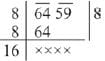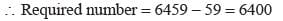Hence 59 must be subtracted.

QUESTION: 4

By what least number 384 be multiplied 50 that the product may be a perfect square?

Solution: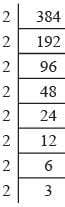∴ 384 = 2 × 2 × 2 × 2 × 2 × 2 × 2 × 2 × 3

∴ Required number = 6

QUESTION: 5

What is the smallest number of four digits which is a perfect square?

Solution:

Consider option (b)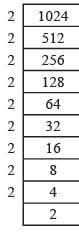Here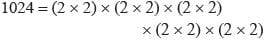Clearly 1024 is the smallest number of four digits which is a perfect square.

QUESTION: 6

What is the greatest number of four digits which is a perfect square?

Solution:

Greatest number of four digits = 9999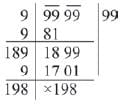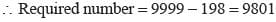QUESTION: 7

Find the least number which must be added to 2292 to make it a perfect square.

Solution: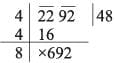2292 + 12 = 2304 which is a perfect square.

QUESTION: 8

Find the smallest number by which 557568 must be divided so that it become a perfect square.

Solution:
QUESTION: 9

Find the smallest number by which 396 must be multiplied so that the product becomes a perfect square?

Solution:

396 = 2 × 2× 3 × 3 × 11
To make a perfect square, 11 is multiplied to 396.

QUESTION: 10

What is the least number which must be added to 6203 to obtain a perfect square ?

Solution: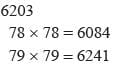6241 – 6203 = 38
38 must be added to 6203 to make a perfect square.

QUESTION: 11

Find the smallest square number that is divisible by each of the number 8, 15 and 20.

Solution:

Here LCM of 8, 15, 20 = 120. and
120 = 2  × 2 × 2 × 3 × 5
To make 120 a perfect square, it must be multiplied by 2 × 3 ×5 = 30
120 × 30 = 3600

QUESTION: 12

What is the least number that must be added to 1300 so as to get a perfect square?

Solution:

36 × 36 = 1296
37 × 3 7 = 1369
69 must be added to make 1300 as perfect square.

QUESTION: 13

What is the length of the diagonal of a square whose perimeter is equal to the perimeter of an equilateral triangle of side 4 cm?

Solution:

Perimeter of an equilateral triangle
= 4 + 4 + 4 = 12 cm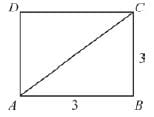Perimeter of square = 12 cm ⇒ 4 × side = 12 ⇒ Side =  3 cm.
∴    Length of diagonal =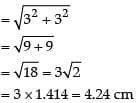QUESTION: 14

The product of two positive numbers is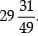and one of them is three times the other. Find the larger number.

Solution:

Let the numbers are x and 3x.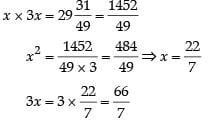QUESTION: 15

The area of a rectangular field whose length is three times its breadth is 348 m2. What is the perimeter of the field?

Solution:

∴ Length = 3x m
Now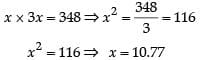∴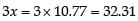Perimeter = 2 (10.77 + 32.31) = 86.16 m

QUESTION: 16

Which of the following is not a Pythagorean triplet?

Solution:

Here 32 + 42 = 52
62 + 82 = 102
22 + 32 ≠ 42
122 + 352 = 372
∴ (c) is not a Pythagorean triplet.

QUESTION: 17

The area of a square field is 60025 m2. A man cycles along its boundary at 18 km/hour. In how much time will he return to the starting point?

Solution:

Speed of cycle = 18 km/hour
18 x 5/18
5m/sec
Area of square = 60025
Side of square =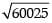= 245 m
Perimeter of square = 245 × 4 m
∴    Time taken =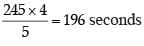QUESTION: 18

What is the length of each side of a square whose area is equal to the area of a rectangle of length 13.6 m and breadth 3.4 meters?

Solution:

Area of square = Area of rectangle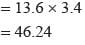∴ Side of square =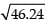= 6.8 m

QUESTION: 19

The perimeter of a square field is 76m. What is its area?

Solution:

Perimeter of square = 76
⇒    4 × side = 76 ⇒ side = 76/4 = 19
∴ Area of square = 192 = 361 m2

QUESTION: 20

Which of the following is the square of an even number?

Solution:

Here 729 = 272, 324 = 182, 441 =  212, 625 = 252
or
Square of an odd number is an odd number.Use Code STAYHOME200 and get INR 200 additional OFF Use Coupon Code